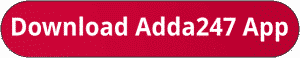Latest Banking jobs   »   IBPS po pre quant

# Quantitative Aptitude Quiz For IBPS RRB PO/Clerk Prelims 2023 -16th July

Q1. Harish covers a distance of 720 km in 8 hours. If speed of Shivam is 4/3 rd of the speed of Harish, then find time taken by Shivam to cover 3/4 th of the distance that is covered by Harish.
(a) 5 hours
(b) 3.6 hours
(c) 4 hours
(d) 4.5 hours
(e) 5.2 hours

Q2. A and B invests a total amount of Rs 10000 in two schemes respectively for two years. A invests at rate of 10% per annum at CI while B invests at rate of 12.5% at SI. If interest earned by B is Rs 660 more than A, then find amount invested by B.
(a) Rs 4000
(b) Rs 5500
(c) Rs 6000
(d) Rs 6500
(e) Rs 5000

Q3. A boatman starts covering distance from a point in downstream. After covering a distance of 180 km, the boatman returns to initial point. If the speed of the boat in still water is 36 kmph and speed of stream is 9 kmph. Find the total time taken by boatman during his whole trip.
(a) 9 hour 30 min
(b) 10 hour 40 min
(c) 8 hour 20 min
(d) 9 hour 50 min
(e) 10 hour 30 minQ5. There are 4 consecutive even numbers. If sum of first three numbers is 108, then calculate the product of smallest and largest number.
(a) 1260
(b) 1292
(c) 1280
(d) 1360
(e) 1428

Directions (6 – 10): In each of these questions, two equations (I) and (II) are given. You have to solve both the equations and answer the following questions.
(a) x > y
(b) x < y
(c) x ≥ y
(d) x ≤ y
(e) x = y or no relation.Solutions. .                              .

## FAQs

### What is the selection process of IBPS RRP PO?

The Selection Process of IBPS RRB PO consists of Prelims, Mains and Interview

#### Congratulations!Union Budget 2023-24: Free PDF